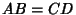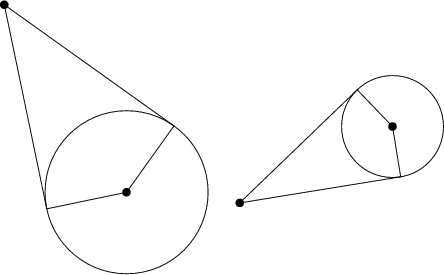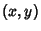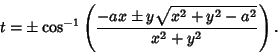## Circle Tangents

There are four Circles that touch all the sides of a given Triangle. These are all touched by the Circle through the intersection of the Angle Bisectors of the Triangle, known as the Nine-Point Circle.Given the above figure,, sinceBecause, it follows that.The line tangent to a Circle of Radiuscentered atthroughcan be found by solving the equationgivingTwo of these four solutions give tangent lines, as illustrated above.

See also Kissing Circles Problem, Miquel Point, Monge's Problem, Pedal Circle, Tangent Line, Triangle

References

Dixon, R. Mathographics. New York: Dover, p. 21, 1991.

Honsberger, R. More Mathematical Morsels. Washington, DC: Math. Assoc. Amer., pp. 4-5, 1991.